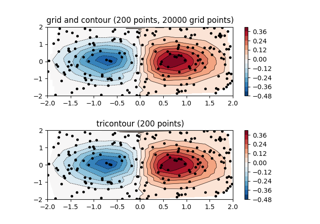matplotlib.pyplot.subplots_adjust(left=None, bottom=None, right=None, top=None, wspace=None, hspace=None)[source]

Adjust the subplot layout parameters.

Unset parameters are left unmodified; initial values are given by `rcParams["figure.subplot.[name]"]`.

Parameters
leftfloat, optional

The position of the left edge of the subplots, as a fraction of the figure width.

rightfloat, optional

The position of the right edge of the subplots, as a fraction of the figure width.

bottomfloat, optional

The position of the bottom edge of the subplots, as a fraction of the figure height.

topfloat, optional

The position of the top edge of the subplots, as a fraction of the figure height.

wspacefloat, optional

The width of the padding between subplots, as a fraction of the average Axes width.

hspacefloat, optional

The height of the padding between subplots, as a fraction of the average Axes height.

## Examples using `matplotlib.pyplot.subplots_adjust`¶Contour plot of irregularly spaced data¶